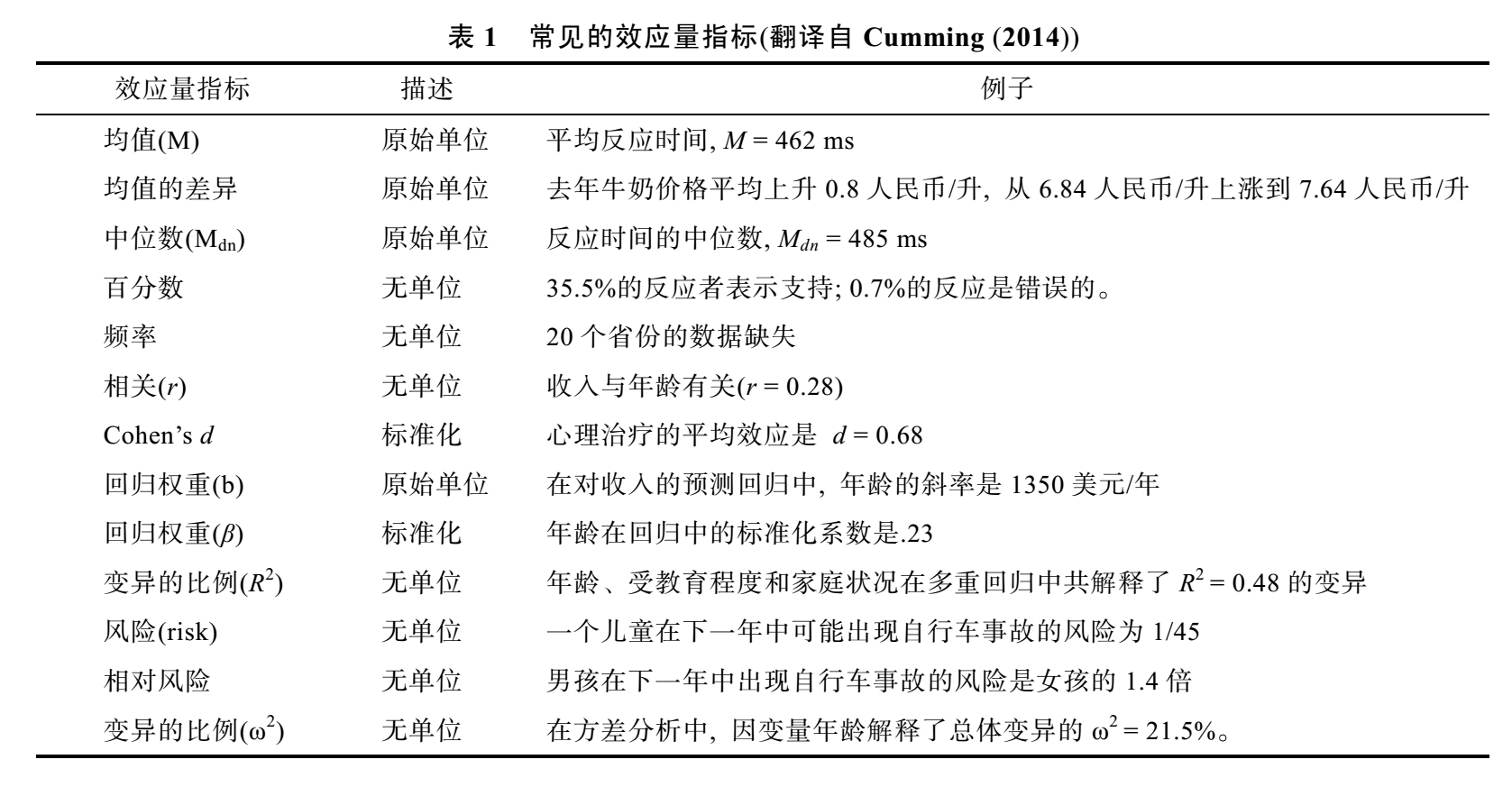## 假设检验的术语与概念

##### 定义：实验检验力(the power of an experiment)

β定义为犯II型错误的概率，β=1-power。

## 假设检验的过程

1. 重复测量设计(repeat measures design)

这种设计最基本的形式只包含两种条件：实验条件控制条件。这两种条件中除了自变量外，其他因素要尽可能相同。是为控制变量法。

2. 提出备择假设$H_1$与相应的虚无假设$H_0$

任何实验中解释结果都要权衡两种假设：备择假设(Alternative hypothesis)与虚无假设(Null hypothesis)。

3. 检验虚无假设$H_0$：选择、计算并检验合适的统计量

假设自变量无效，即虚无假设为真，样本是从虚无假设总体中随机抽取的。

计算统计量得到该结果或更极端结果的发生概率p。

然后根据α水平进行决策，如果p≤α则拒绝$H_0$，否则保留$H_0$。

## 假设检验的结果

$H_0$为真（随机因素）$p_1$ 正确决策，真阴性，TN， (1-α) I型错误，FP，错误的拒绝了$H_0$(α)
$H_0$为假（自变量有效）$p_2$ II型错误，FN，错误的保留了$H_0$(β) 正确决策，真阳性，TP，(power = 1-β)
• α水平确定了犯I型错误的概率水平，β水平确定了犯II型错误的概率水平。
• p值是当你假定没有效应时，当前数据有多大的可能会出现。
• 决策原理是：我们人为地规定一个值（心理学中的0.05，物理学中的0.0000003，正态分布约5σ），假如p值小于这个值，我们就认为可能是有效应的。
• $H0$为真($p_1$)为假$p_2$的概率，我们是不知道的。所能知道的只是当$H_0$为真，即只有随机因素作用时，出现当前结果模式的概率。即$p=P(D^*|H_0)$。不能根据p值推断出$H_0$为真的概率$p_1$。因为以$H_0$为真作为条件时，当前数据模式的概率，不等于以当前数据模式作为条件，$H_0$为真的概率。

$$P(D^|H_0) \ne P(H_0|D^)$$

• p> 0.05也不能说明没有效应，有可能是效应比较小，需要更多的样本才能检测出效应。p < 0.05也只能说明自变量是显著的，即这一因素不是由随机因素引起的，有实际效应存在。但显著不等于重要：一个极其微小的差别影响通过海量样本被探测出来显著，很可能没什么卵用。知道某个效应有多大，比知道这个效应存在更有意义，这就引出的效应量的概念。• 如图，假设待检验统计量满足正态分布，左侧为从**虚无假设总体(null hypothesis population)**采样得到的统计量的分布，右侧为自变量有真实效应时从总体采样得到统计量结果的概率分布。。

• 两个统计量的分布都是PDF，曲线与坐标轴成的面积都为1，向两侧无限接近坐标轴。

• $z_{crit}$是根据α设定的决策临界值，它将$H_0$和$H_1$的分布各自划分为两个区域。

• 当自变量没有效果时，保留虚无假设，此时样本实际从$H_0$分布中采样得到：

• 红色拒绝域面积为α，如果$H_0$真的成立的话，结果落入此处的概率非常小。因此当结果落入红色区域时，我们认为小概率实际实际不可能发生，做出拒绝$H_0$，接受$H_1$的决策。但竟然真的因为随机因素产生这么极端的结果，I型错误就发生了，FP，发生概率为α。
• 样本落在白色区域(被盖住一小块)时，决策结果无法拒绝$H_0$。结果为TN，发生概率为1-α。
• 当自变量有实际效果时，拒绝虚无假设，此时样本实际从$H_1$分布采样得到：

• 蓝色区域，代表拒绝了$H_0$，且做出正确的决策：TP，其面积等于检验力(statistic power)，1-β
• 黑色区域，因为结果并不显著，做出了保留了$H_0$的决策，认为自变量没有效果。但实际上是因为随机因素，从$H_1$的分布中抽到了极端的结果，错误的保留了$H_0$，就犯了II型错误，FN，发生概率为β。

## 假设检验的效应量

##### 定义：效应量(effect size)，ES值但总体而言，效应量一般具有三个特性：

• 尺度不变性(scale free)：观测单位发生变化不影响ES。
• 绝对值大小与效应强弱一致：取值应为从0开始的连续变量，当虚无假设为真时，ES理想估计值为0
• 非样本量依赖性：ES指标应较少受样本容量的影响。

#### 效应量的作用

**效应量ES、统计检验力power=(1-β)、α水平，样本量N，这四个变量相互关联，知道其中三个就可以推导出第四个。**由于在心理学研究中alpha水平通常被定为0.05，而统计检验力理论上越高越好，大多数实验的检验力值在40%~60%之间。80%已经非常理想。在这种情况下，α与统计检验力(1-β)都可以设定，所以如果知道效应量，就可以对研究所需要的样本量N进行估计。

• 当样本量N增大时，比如平均数的采样分布方差就会缩小，采样的分布就越高瘦。
• 此时如果α和β保持不变，则所需的效应量变小。
• 如果待检测的效应量ES和决策水平alpha不变，则β减小，检验力提升。

## 假设检验的种类

• 单样本z检验
• 单样本t检验
• 相关样本t检验
• 维尔克松检验
• 符号检验
• 独立样本t检验
• 曼-惠特尼U检验
• 单因素方差分析F检验
• 克拉斯科-沃利斯检验
• 双因素方差分析F检验
• $\chi ^2$检验